Smartick is an advanced online program that teaches kids math and coding in only 15 min. a day
Try it for free!

Jan21

# Digital Manipulative Materials Used by the Smartick Method

It is becoming more and more common to find digital manipulative materials for learning mathematics.

At Smartick we are aware that manipulative and pictorial representations are fundamental for the acquisition of some mathematical content. However, these materials should be organized in order to get the most out of them. In other words, the use of manipulative materials without organization will not produce the expected learning because, in addition to the materials, a proper sequence of tasks is necessary to produce learning.

At Smartick, we have incorporated a large variety of manipulative materials for the different mathematical contents of kindergarten and primary education. Additionally, we organize the work by adjusting the curricular recommendations and learning trajectories of children for the different contents.

In this post, we are going to describe some of the manipulative materials that we use in the Smartick method.

### Rekenrek

The rekenrek abacus was one of the first virtual manipulative materials that we incorporated. As we have explained in other posts, with a rekenrek we work with subitization (counting at a glance), a process that provides visual, instant, and global access to the number.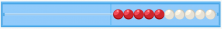### Series

We work with searching for patterns and inductive reasoning from an early age. As explained in this post, discovering, describing, and anticipating what will come next in patterns is an important aspect in mathematics.

In addition, working with patterns develops inductive reasoning, moving from the particular to the general, and is part of the early development of algebraic thought.### Base 10 blocks

It is a didactic resource that allows us to understand and visualize the decimal number system in a concrete way. This manipulative material helps with understanding the positional value, as well as the comprehension of algorithms of arithmetic operations (add, subtract, multiplication, division), the order of units, and the metric system.

This material is made up of pieces that represent the units (small cubes), the tens (bars), the hundreds (plates), and the millions (cubes).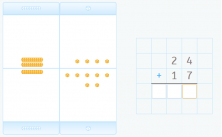### Montessori number cards

The same as the base 10 blocks, they help us to understand the decimal system, putting together, and breaking down numbers. Furthermore, we use them to teach how to read numbers 1 to 9999.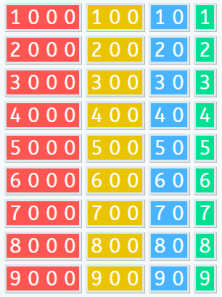### 100 square

The 100 square is a material that helps our students develop numerical sense, in other words, flexible mental calculation. We can also use it as a way to memorize multiplication tables, which we explained in this post.

Additionally, Smartick also uses it to help you work on more complex mathematical concepts like least common multiple, for example.

##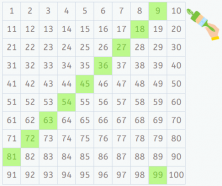### Multiplying fractions through the area model

Although multiplication with Smartick starts as a repetitive sum, soon we see the area model, which is an essential model to work with multiplication. We use it with natural numbers, fractions, and polynomials.

The use of the same model for different concepts helps to expand and develop the mathematical concept.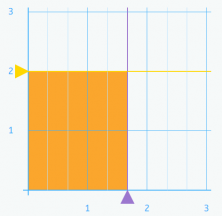### Money

Money is a non-proportional model, which means it does not maintain any size relationship between its parts. However, since it is a material that children are very familiar with, it facilitates the learning and understanding of decimal numbers and their operations.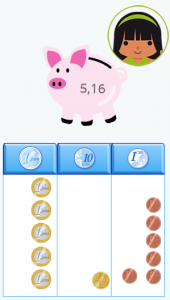### Algebra Tiles

Algebra Tiles are a manipulative material derived from base 10 blocks. It is made up of rectangles: a square with side a, another of side 1, and a rectangle of sides a x 1, etc.

Additionally, it is a system used to represent polynomials, this material allows us to make sense of the factorization process, adding, subtracting, multiplying some polynomials, and solving some equations.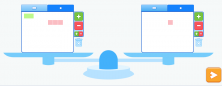All of these tools and manipulative materials are available in Smartick sessions. Sign up and try our method for free, you will love it!

Learn More: